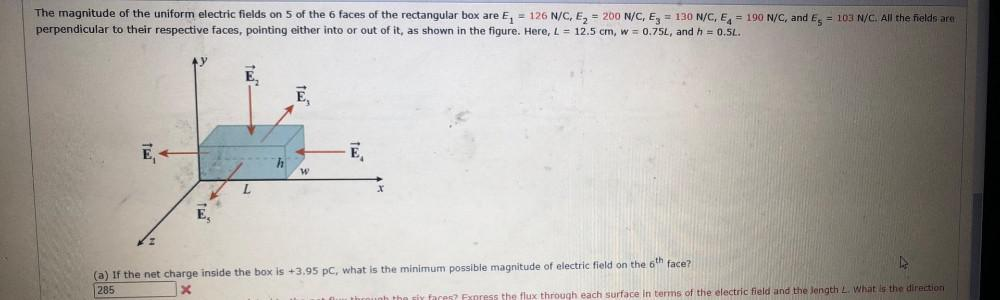Question:

# - The magnitude of the uniform electric fields on 5 of the 6 faces of the rectangular box are E = 126 N/C, E2 = 200 N/C, Ez = 13- The magnitude of the uniform electric fields on 5 of the 6 faces of the rectangular box are E = 126 N/C, E2 = 200 N/C, Ez = 130 N/C, E4 = 190 N/C, and Es = 103 N/C. All the fields are perpendicular to their respective faces, pointing either into or out of it, as shown in the figure. Here, L = 12.5 cm, w = 0.75L, and h = 0.5L. E, Ē, Ē. h W L E, (a) If the net charge inside the box is +3.95 pc, what is the minimum possible magnitude of electric field on the 6th face? 285 X ess the flux through each surface in terms of the electric field and the length L. What is the direction